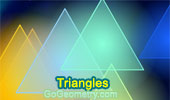# Triangles: Theorems and Problems 29Triangle, Medians, Six Circumcenters Concyclic. Dynamic Geometry.
Step-by-Step construction, Manipulation, and animation.
Prove proposition.

Trapezoid, Triangle, Diagonals, Midpoints. Dynamic Geometry.
Step-by-Step construction, Manipulation, and animation.
Prove proposition.

Triangle: Incircle, Perpendicular, Angle Bisector. Dynamic Geometry.
Step-by-Step construction, Manipulation, and animation.
Prove proposition.

Proposed Problem 133. Triangle, Angle Bisectors, Collinear Points.

Proposed Problem 132.
Triangle, 60 degree, Orthocenter, Congruence, Midpoint.

Proposed Problem 131. Van Aubel Theorem, Concurrent Cevians, Sum of Ratios.

Proposed Problem 130. Triangle, Concurrent Cevians, Sum of Ratios.

Proposed Problem 129. Triangle, Concurrent Cevians, Sum of Ratios.

Proposed Problem 128. Incenter of a Triangle, Angle Bisectors, Sum of Ratios.

Go to Page: Previous | 1 | 2 | 3 | 4 | 5 | 6 | 7 | 8 | 9 | 10 | 11 | 12 | 13 | 14 | 15 | 16 | 17 | 18 | 19 | 20 | 21 | 22 | 23 | 24 | 25 | 26 | 27 | 28 | 29 | 30 | 40 | Next

 Home | Search | Geometry | Polygon | Email | By Antonio Gutierrez